Next: Uniqueness Up: tr02cb1 Previous: Introduction

# Probabilistic ICA model

Similar to the square noise-free case, the probabilistic ICA model is formulated as a generative linear latent variables model. It is characterised by assuming that the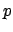-variate vector of observations is generated from a set of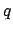statistically independent non-Gaussian sources via a linear instantaneous mixing process corrupted by additive Gaussian noise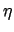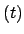: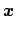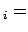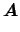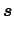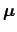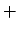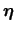(2)

Here,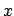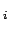denotes the-dimensional column vector of individual measurements at voxel location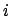,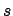denotes the- dimensional column vector of non-Gaussian source signals contained in the data anddenotes Gaussian noise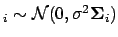. We assume that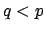, i.e. that there are fewer source processes than observations in time. The covariance of the noise is allowed to be voxel dependent in order to allow for the vastly different noise covariances observed in different tissue types [Woolrich et al., 2001].

The vector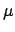defines the mean of the observationswhere the indexis over the set of all voxel locations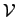and the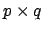matrix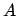is assumed to be non-degenerate, i.e. of rank. Solving the blind separation problem requires finding a linear transformation matrix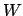such that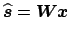is a good approximation to the true source signals.

The PICA model is similar to the standard GLM with the difference that, unlike the design matrix in the GLM, the mixing matrixis no longer pre-specified prior to model fitting but will be estimated from the data as part of the model fitting. The spatial source signals correspond to parameter estimates in the GLM with the additional constraint of being statistically independent.

The model of equation 2 is closely related to Factor Analysis (FA) [Bartholomew, 1987]. There, the sources are assumed to have a Gaussian distribution and the noise is assumed to have a diagonal covariance matrix. In Factor Analysis, the sources are known as common factors andis a vector of random variables called specific factors. In FA the assumption of independence between the individual source processes reduces to assuming that sources are mutually uncorrelated.

SubsectionsNext: Uniqueness Up: tr02cb1 Previous: Introduction
Christian F. Beckmann 2003-08-05